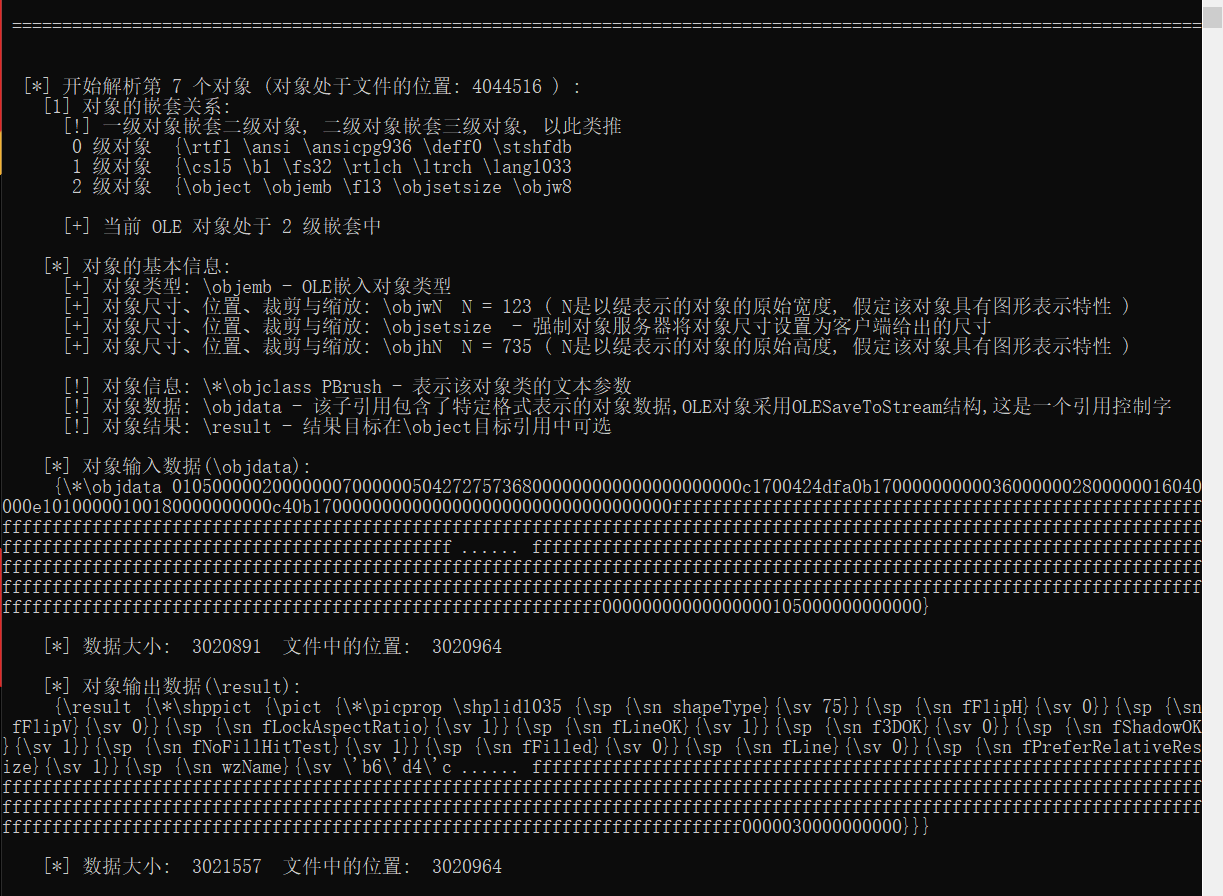### python读取rtf文件_基于 RTF specification v1.7 的 RTF 文件解析及 OLE 对象提取（使用 Python 开发）…

0x01 Office RTF 文件介绍

RTF 文件也称富文本格式（Rich Text Format, 一般简称为 RTF），意为多文本格式是由微软公司开发的跨平台文档格式。大多数的文字处理软件都能读取和保存 RTF 文档。RTF 是一种非常流行的文件结构，很多文字编辑器都支持它，vb 等开发工具甚至还提供了 richtxtbox 的控件。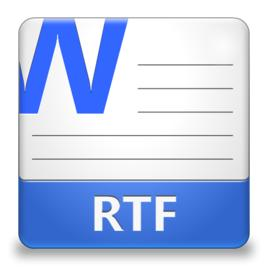RTF 和 DOC 文件一样，都属于 Microsoft Office 的范畴，和 DOC 文件类似，RTF 文件也可以进行文字编辑操作，甚至是插入 OLE 对象来增强文件的互操作性，例如公式编辑器、嵌入式 PPT 文件、嵌入式 DOC 文档、WordPad Document 以及位图文件等等。之后为了方便对 DOC 的兼容性操作，提供了将 RTF 文件嵌入 DOC 文档的功能。

0x02 根据 RTF Specification Version 1.7 分析 RTF 文件

RTF Specification Version 1.7 是 RTF 的文件规范，就如下图所示：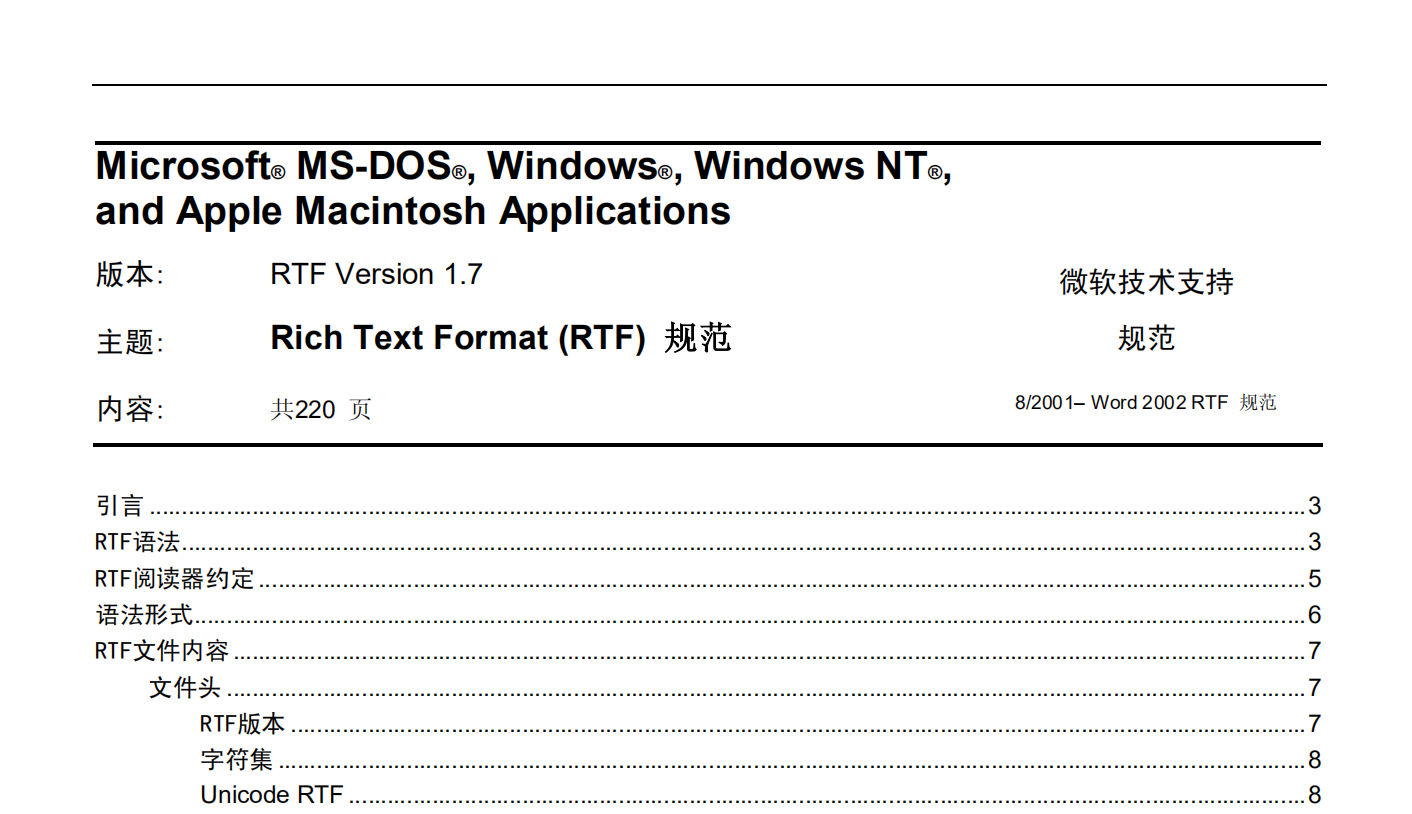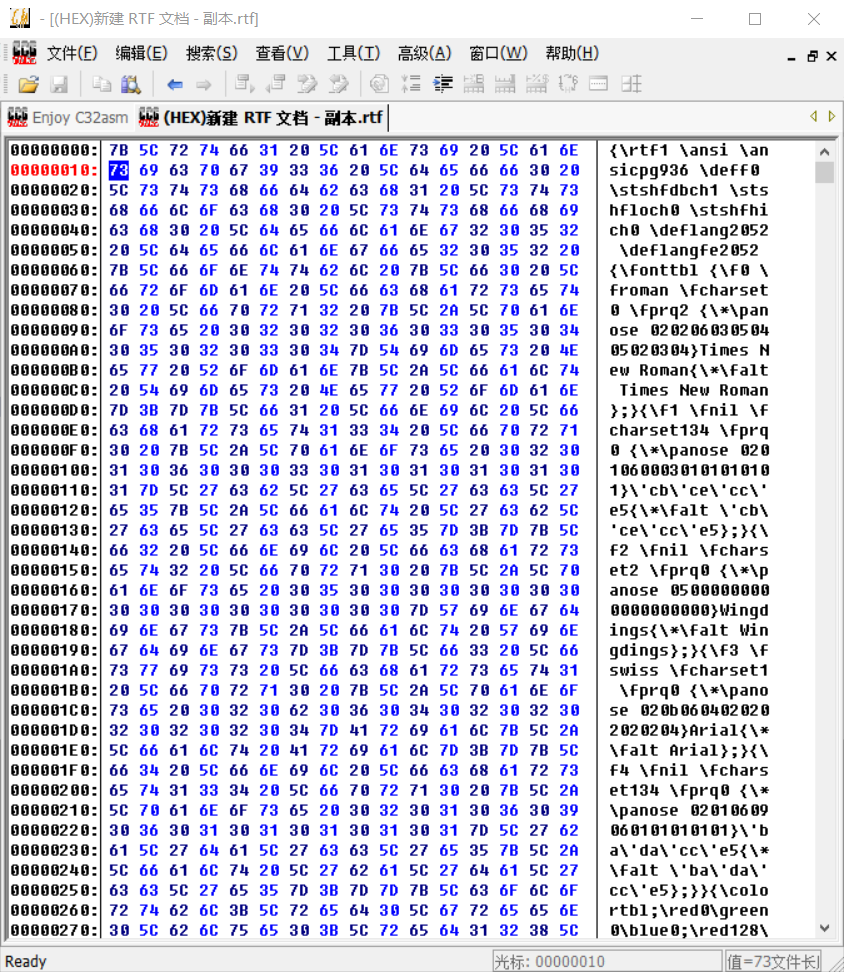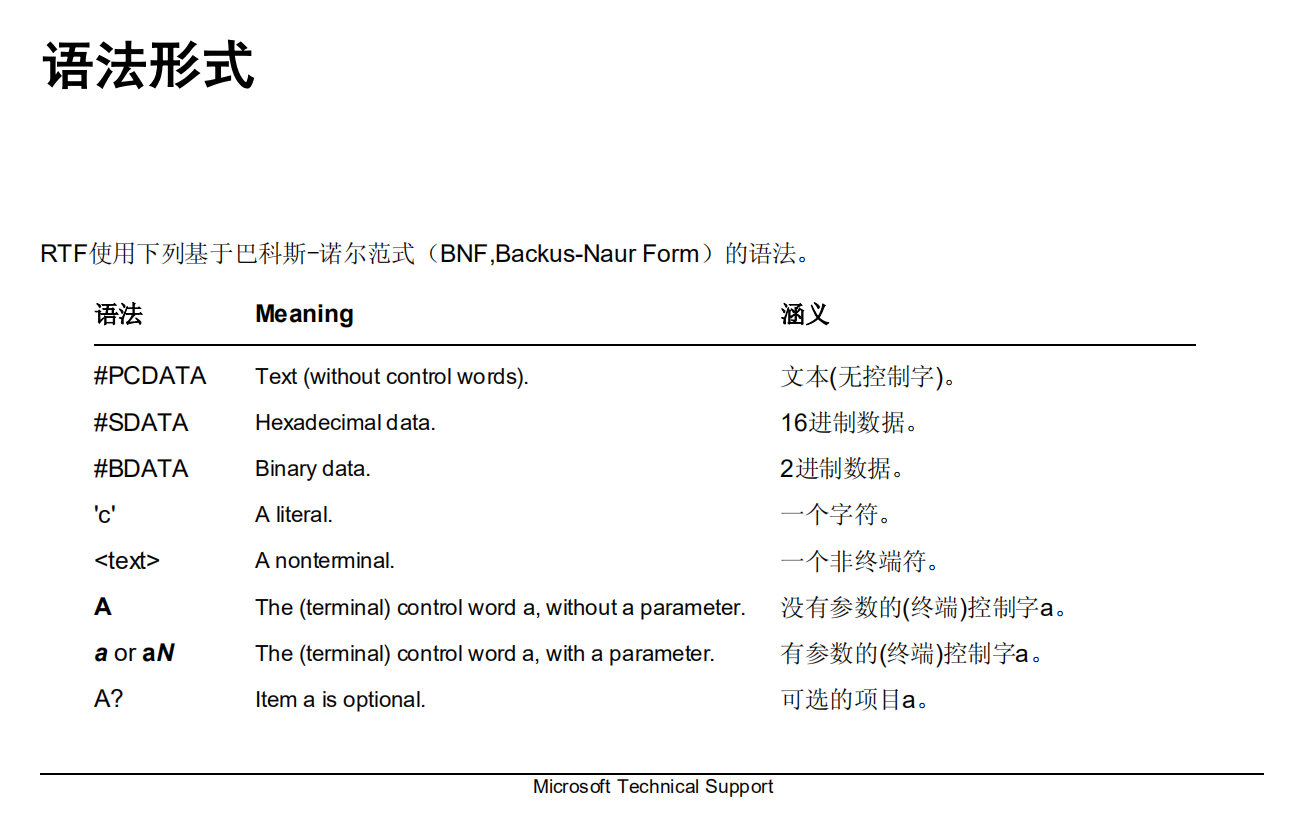0x03 制定解析算法

a) 组平衡算法

# 组平衡判断

def balance(data, seek):

local_1 = 0;

for i in data:

if(i == ‘{‘):

local_1 += 1;

if(i == ‘}’):

local_1 -= 1;

local_1 += seek;

if(local_1 == 0):

return True;

return False;

b) 控制字和组分离算法

# 核心函数, 将数据解析, 得出控制字和组

def rtfAnalysis(data):

# 对数据进行组平衡判断

if(balance(data, 0) == False):

print(“[-] 解析出错, RTF 组格式损坏”);

exit(0);

# – 组控制字解析算法:

# 根据 RTF 1.7 文档规范以及解析的功能, 对 RTF 数据中的 \ 、 \\ 、 { 、 } 字符对文件进行分割, 达到分离

# 控制字和组的目的, 之后使用 python 独有的切片操作, 将分割后的字符储存在集合中, 最后返回集合, 达到分离

# 并解析 RTF 数据的目的, 本算法可能在效率上不及压入弹出算法

local_1 = 0; local_2 = 0; local_3 = 0; listgroup1 = []; listgroup2 = [];

for i in data:

if(i == ‘\\’ and local_3 == 0):

if(data[local_1 + 1] != ‘*’ and data[local_1 – 1] != ‘*’ ):

listgroup1.append(i); listgroup2.append(local_1);

elif(data[local_1 + 1] == ‘*’):

listgroup1.append(i); listgroup2.append(local_1);

if(i == ‘{‘ or i == ‘}’):

local_3 = 1;

if(i == ‘{‘):

if(local_2 == 0):

listgroup1.append(i); listgroup2.append(local_1);

local_2 += 1;

if(i == ‘}’):

local_2 -= 1;

if(local_2 == 0):

listgroup1.append(i); listgroup2.append(local_1);

local_3 = 0;

local_1 += 1;

local_1 = 0; local_2 = 0; local_3 = 0; listgroup3 = {};

for list1 in listgroup1:

if(local_1 < len(listgroup2) – 1):

if(list1 == ‘\\’):

listgroup3[listgroup2[local_1]] = data[listgroup2[local_1]:listgroup2[local_1 + 1]].strip();

elif(list1 == ‘{‘):

listgroup3[listgroup2[local_1]] = data[listgroup2[local_1]:listgroup2[local_1 + 1] + 1];

else:

if(list1 != ‘}’):

listgroup3[listgroup2[local_1]] = data[listgroup2[local_1]:len(data) + 1].strip();

local_1 += 1;

return listgroup3;

c) 组嵌套和控制字查找算法

# 查找特定的组, 记录其组嵌套关系, 并返回完整的组

def searchGroup(data, findStr):

WSize = len(findStr); WSPosition = [];

for i in range(len(data)+1):

if(i + WSize == len(data) – 3):

break;

CWData = data[i:i + WSize];

if(CWData == findStr):

WSPosition.append(i);

local_1 = 0; local_2 = 0; dict1 = {};

for m in WSPosition:

grouplist = [];

for n in data[0:m]:

if(n == ‘{‘):

grouplist.append(local_1);

if(n == ‘}’):

grouplist.pop();

local_1 += 1;

dict1[m] = grouplist;

local_1 = 0;

return dict1;

0x04 制定脚本功能

a) 列出所有组和控制字符

b) 对文件进行基本解析

c) 解析文件中的 ole 对象

0x05 编写脚本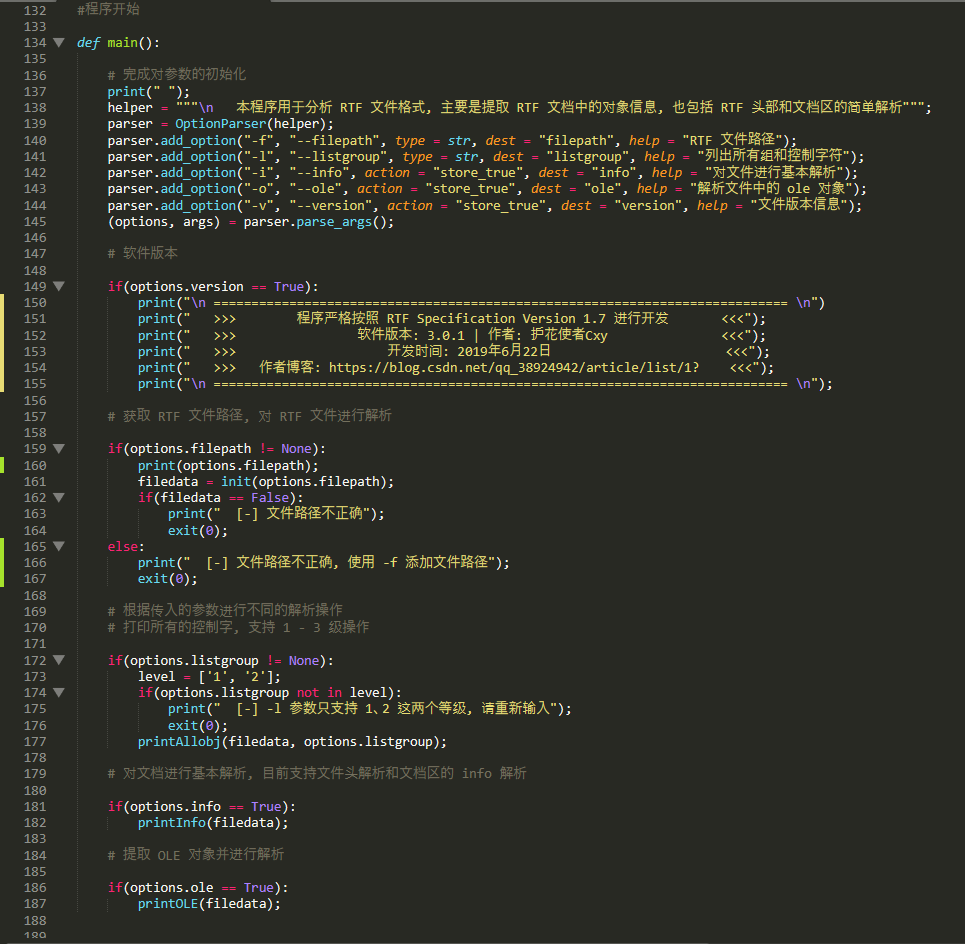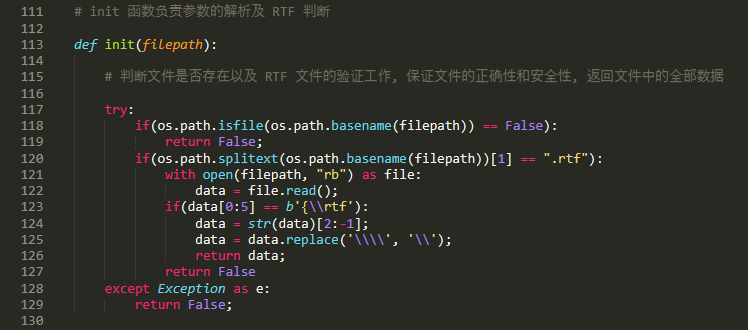a) 使用 printAllobj() 函数打印所有的组和控制字符

# 根据解析的结果打印组和控制字

def printAllobj(data, level):

# 该函数主要为了打印出二级和三级列表下的组或控制字, 完成对 RTF 文件的基本解析

print(” [+] 开始解析: “);

print(” [*] 注: \”>\” 代表一级组列表下的或控制字 ; \”>>\” 代表二级列表下的组或控制字 ; \”>>>\” 代表三级列表下的组或控制字”);

print(” “)

group = rtfAnalysis(data);

local_1 = 0; local_2 = 0; local_3 = 0;

for i in group:

local_1 += 1;

if(group[i] == ‘{‘):

print(” >>>”, group[i][0:30], “……”, group[i][-30:]);

listgroup = rtfAnalysis(group[i][1:-1]);

for m in listgroup:

local_2 += 1;

if(listgroup[m] == ‘{‘):

print(” >>”, listgroup[m][0:30], “……”, listgroup[m][-30:]);

if(level == “2”):

listgroup1 = rtfAnalysis(listgroup[m][1:-1]);

for n in listgroup1:

local_3 += 1;

if(listgroup1[n] == ‘{‘):

print(” >”, listgroup1[n][0:30], “……”, listgroup1[n][-30:]);

else:

if(listgroup1[n] == ‘\”):

pass

else:

print(” >”, listgroup1[n]);

else:

if(listgroup[m] == ‘\”):

pass

else:

print(” >>”, listgroup[m]);

else:

if(group[i] == ‘\”):

pass

else:

print(” >>>”, group[i]);

print(“\n [+] 解析完毕”);

print(” [+] 结果:”);

print(” [>] 一级对象或控制字总个数:”, local_1, “|”, “二级对象或控制字总个数:”, local_2, “|”, “三级对象或控制字总个数:”, local_3);

print(” [>] RTF 对象格式完好, 不存在缺失状况\n”);

print(” [+] 如需要更详细的了解某些控制字和组, 可以参照 RTF 规范文档”);

b) 使用 printInfo() 函数对文档进行基本解析

# 打印 RTF 文件基本信息

def printInfo(data):

group1 = rtfAnalysis(data);

if(group1[0:5] != “{\\rtf”):

return False;

group2 = rtfAnalysis(group1[1:-1]);

group1 == {};

for i in group2:

if(group2[i] == ‘{‘):

break;

group1[i] = group2[i];

RTF_characterSet = {“\\ansi”:”ANSI (默认)”, “\\mac”:”Apple Macintosh”, “\\pc”:”IBM PC code page 437″, “\\pca”:”IBM PC code page 850″};

RTF_deffont = {“\\stshfdbch”:”远东字符”, “\\stshfloch”:”ASCII字符”, “\\stshfhich”:”High-ANSI字符”, “\\stshfbi”:”Complex Scripts (BiDi)字符”};

RTF_group = {“\\fonttbl”:”字体表”, “\\filetbl”:”文件表”, “\\colortbl”:”颜色表”, “\\stylesheet”:”样式表”, “\\listtable”:”编目表”, ” \\*\\rsidtbl”:”RSID”, “\\*\\generator”:”生成器”};

# 开始解析 RTF 头部信息

print(” > 开始解析:\n\n # 文件头部信息:\n”);

# 解析 RTF 版本

list1 = [];

for i in group1:

list1.append(group1[i]);

rtfN = list1;

rtfVersion = rtfN[rtfN.find(“\\rtf”) + len(“\\rtf”):];

print(” [+] RTF 版本号:”, rtfVersion);

# 解析字符集

local_1 = 0;

for m in list1:

for n in RTF_characterSet:

if(m == n):

print(” [+] 字符集:”, n[1:]);

local_1 = 1;

if(local_1 == 0):

print(” [+] RTF 文件缺少字符集”);

# 解析默认 Unicode RTF

for m in list1:

if(m.find(“\\ansicpg”) != -1):

print(” [+] Unicode RTF(\\ansicpg):”, m[m.find(“\\ansicpg”) + len(“\\ansicpg”):]);

# 解析控制字 deffont

local_1 = 0;

for m in list1:

for n in RTF_deffont:

if(m.find(n) != -1):

print(” [*]”, n[1:], “:”, m[m.find(n) + len(n):]);

local_1 = 1;

if(local_1 == 0):

print(” [+] RTF 文件缺少 deffont 控制字”);

# 解析其他文件头部中存在的可被解析的组对象

print(“\n [+] RTF 文件头部被解析出的其他对象: \n”);

for m in group2:

for n in RTF_group:

if(group2[m].find(n) != -1):

print(” [+]”, n,”(“, RTF_group[n], “)”);

print(” “);

# 开始解析 RTF 文档区信息

c) 使用 printOLE() 函数解析 RTF 文件的 OLE 对象

# 解析 RTF 文件的 OLE 对象

def printOLE(data):

olestr = “\\object”;

if(balance(data, 0) == False):

print(“[-] RTF 文件中的组不平衡, 文件可能损坏, 但不影响分析”);

dict1 = searchGroup(data, olestr);

# 开始解析 RTF 文件中的 OLE 对象

print(“# 开始解析 RTF 文件中的 OLE 对象…\n”);

# 打印 RTF 文件中的对象个数

count = 0;

for i in dict1:

count += 1;

print(” [+] RTF 文件中的 OLE 对象个数:”, count);

# 根据对象的首位置取出完整的对象

count = 1; count1 = 0; ole = {};

for m in dict1:

olestart = dict1[m][-1];

olend = olestart;

for n in data[olestart:]:

if(n == ‘{‘ or n == ‘}’):

if(n == ‘{‘):

count += 1;

if(n == ‘}’):

count -= 1;

if(count == 1):

break;

olend += 1;

ole[m] = data[olestart:olend + 1];

# 打印对象的嵌套关系和基本信息

obj_objtype = {“\\objemb”:”OLE嵌入对象类型”, “\\objlink”:”OLE链接对象类型”, “\\objautlink”:”OLE自动链接对象类型”, “\\objsub”:”Macintosh版管理签署对象类型”, “\\objpub”:”Macintosh版管理发布对象类型”, “\\objicemb”:”MS Word for Macintosh可安装命令（IC）嵌入对象类型”, “\\objhtml”:”超文本标记语言（HTML）控件对象类型”, “\\objocx”:”OLE控件类型”};

obj_objmod = {“\\linkself”:”该对象为同一文档中的另一部分”, “\\objlock”:”锁定对象的所有更新操作”, “\\objupdate”:”强制对象在显示之前更新”, “\\*\\objclass”:”表示该对象类的文本参数”, “\\objname”:”表示该对象名称的文本参数”, “\\objtime”:”列出对象最后更新的时间”};

obj_objinfo = {“\\objhN”:”N是以缇表示的对象的原始高度, 假定该对象具有图形表示特性”, “\\objwN”:”N是以缇表示的对象的原始宽度, 假定该对象具有图形表示特性”, “\\objsetsize”:”强制对象服务器将对象尺寸设置为客户端给出的尺寸”, “\\objalignN”:”N是以缇表示的应该对齐制表位的对象的左缩进距离, 用于正确放置公式编辑器方程”, “\\objtransyN”:”是以缇表示的对象应该参考于基线垂直移动的距离,用于正确放置数学方程式”, “\\objcroptN”:”N是以缇表示的顶端裁剪值”, “\\objcropbN”:”N是以缇表示的底端裁剪值”, “\\objcroplN”:”N是以缇表示的左裁剪值”, “\\objcroprN”:”N是以缇表示的右裁剪值”, “\\objscalexN”:”N是水平缩放百分比”, “\\objscaleyN”:”N是垂直缩放百分比”};

obj_objdata = {“\\objdata”:”该子引用包含了特定格式表示的对象数据,OLE对象采用OLESaveToStream结构,这是一个引用控制字”, “\\objalias”:”该子引用包含了Macintosh编辑管理器发行对象的别名记录,这是一个引用控制字”, “\\objsect”:”该子引用包含了Macintosh编辑管理器发行对象的域记录,这是一个引用控制字”};

obj_objres = {“\\rsltrtf”:”如果可能, 强制结果为RTF”, “\\rsltpict”:”如果可能, 强制结果为一个Windows图元文件或者MacPict图片格式”, “\\rsltbmp”:”如果可能, 强制结果为一个位图”, “\\rslttxt”:”如果可能, 强制结果为纯文本”, “\\rslthtml”:”如果可能, 强制结果为HTML”, “\\rsltmerge”:”无论获取任何新的结果均使用当前结果格式”, “\\result”:”结果目标在\\object目标引用中可选”};

count = 1; count2 = 1;

for k in dict1:

print(“\n ======================================================================================================================= \n”);

print(” [*] 开始解析第”, count, “个对象 (对象处于文件的位置:”, k,”) : “);

print(”  对象的嵌套关系:”);

print(” [!] 一级对象嵌套二级对象, 二级对象嵌套三级对象, 以此类推”);

for i in dict1[k]:

print(” “, count2, “级对象 “, data[i:i + 40]);

count2 += 1;

print(“\n [+] 当前 OLE 对象处于”, count2 – 1, “级嵌套中”);

count2 = 0;

# 打印对象基本信息

print(“\n [*] 对象的基本信息:”);

CWord = rtfAnalysis(ole[k][1:-1]);

for m in CWord:

# 解析对象类型

for n in obj_objtype:

if(CWord[m] == n):

print(” [+] 对象类型:”, n, “-“, obj_objtype[n]);

# 解析对象信息

for n in obj_objmod:

if(CWord[m].find(n) != -1):

if(CWord[m].find(“\\*\\”) != -1):

print(“\n [!] 对象信息:”, n, CWord[m].split()[:-1], “-“, obj_objmod[n]);

else:

print(“\n [!] 对象信息:”, n, “-“, obj_objmod[n]);

# 解析对象尺寸、位置、裁剪与缩放

for n in obj_objinfo:

if(n.find(“N”) != -1):

ki = n[0:-1];

seek = CWord[m].find(ki);

if(seek != -1):

if(n.find(“N”) != -1):

print(” [+] 对象尺寸、位置、裁剪与缩放:”, n, ” N =”, CWord[m][len(n):], “(“, obj_objinfo[n], “)”);

else:

print(” [+] 对象尺寸、位置、裁剪与缩放:”, n, CWord[m][len(n):], “-“, obj_objinfo[n]);

# 对象数据

for n in obj_objdata:

if(CWord[m].find(n) != -1 and CWord[m] == “{“):

print(” [!] 对象数据:”, n, “-“, obj_objdata[n]);

# 对象结果

for n in obj_objres:

if(CWord[m].find(n) != -1):

print(” [!] 对象结果:”, n, “-“, obj_objres[n]);

# 打印对象数据

length = 0;

print(“\n [*] 对象输入数据(\\objdata):”);

for i in CWord:

if(CWord[i].find(“\\objdata”) != -1):

length = len(CWord[i]);

print(” “, CWord[i][0:400], “……”, CWord[i][-400:]);

print(“\n [*] 数据大小: “, length, ” 文件中的位置: “, i);

length = 0;

print(“\n [*] 对象输出数据(\\result):”);

for i in CWord:

if(CWord[i].find(“\\result”) != -1):

length = len(CWord[i]);

print(” “, CWord[i][0:400], “……”, CWord[i][-400:]);

print(“\n [*] 数据大小: “, length, ” 文件中的位置: “, i);

count += 1;

0x05 脚本测试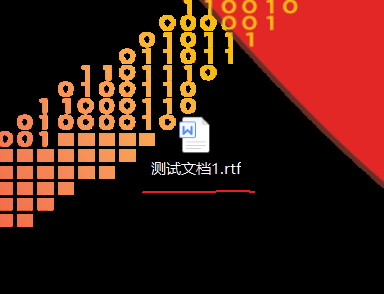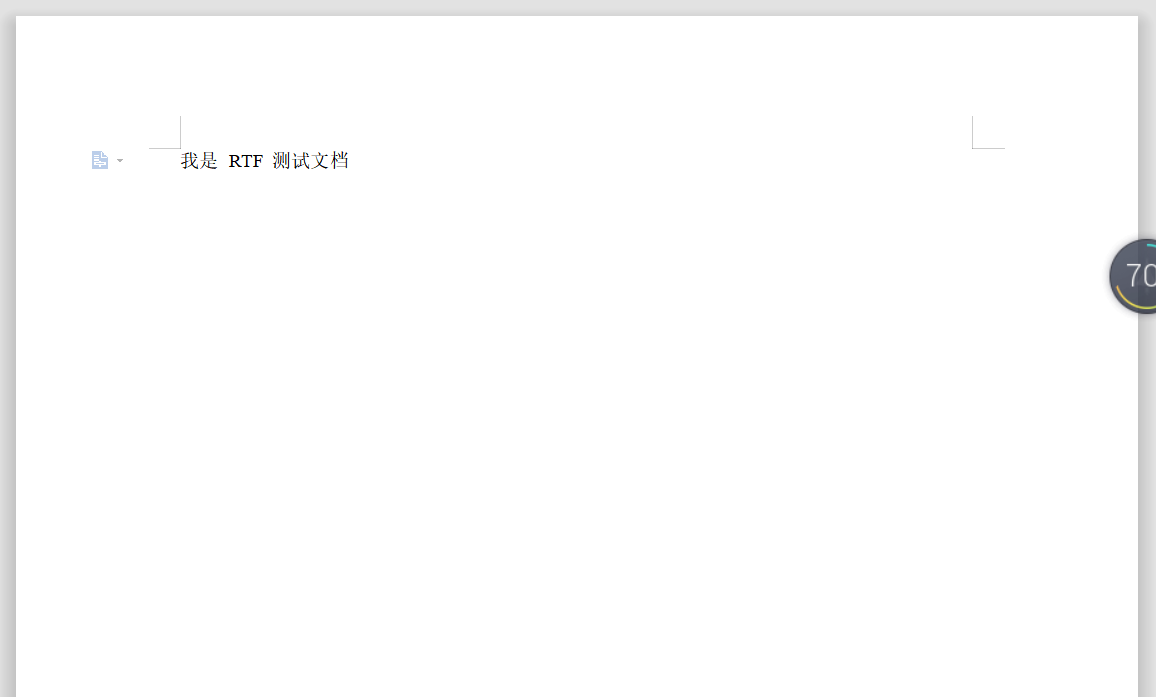a) 打印所有组和控制字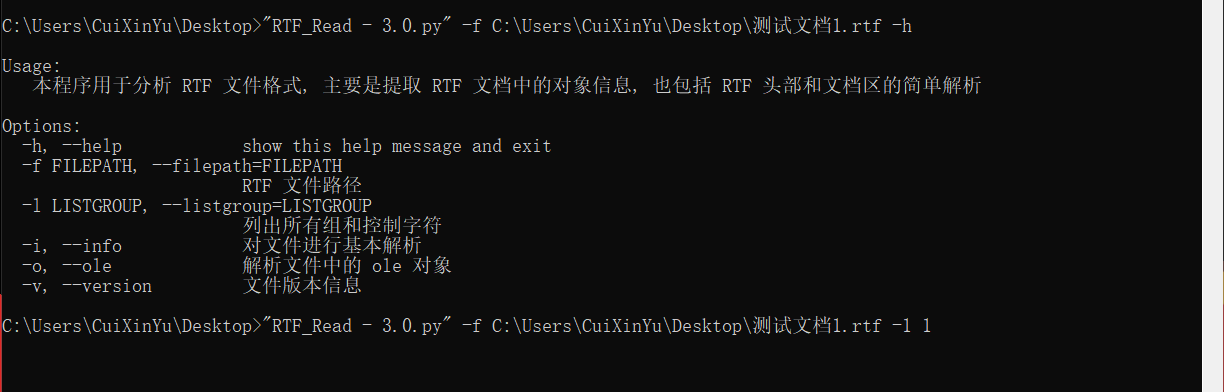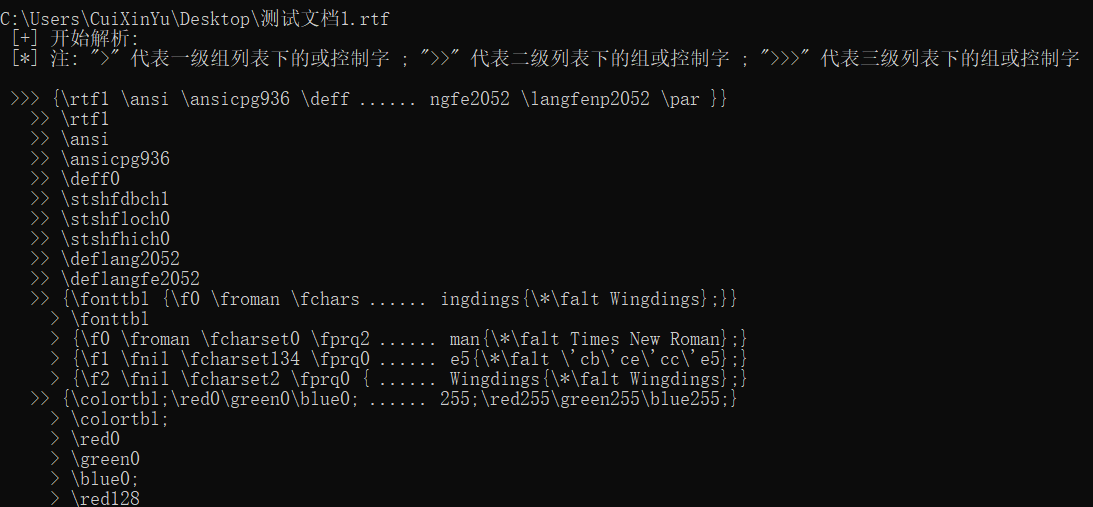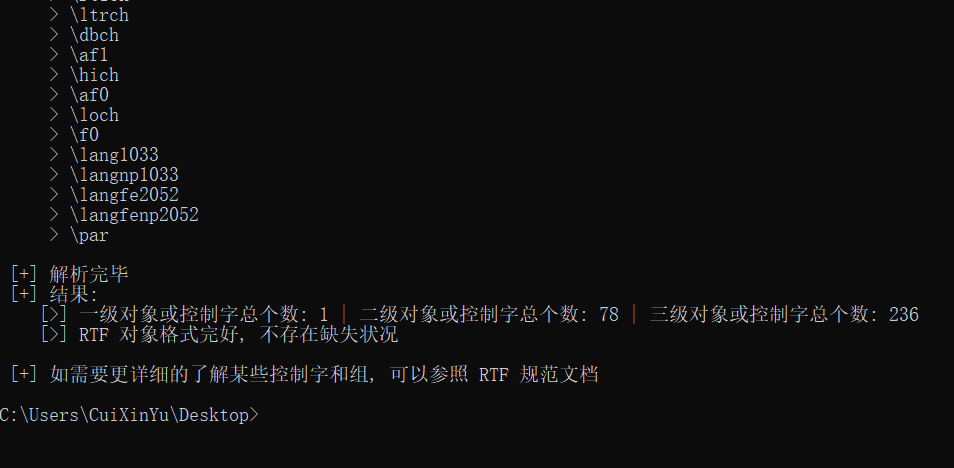b) 对文件进行基本解析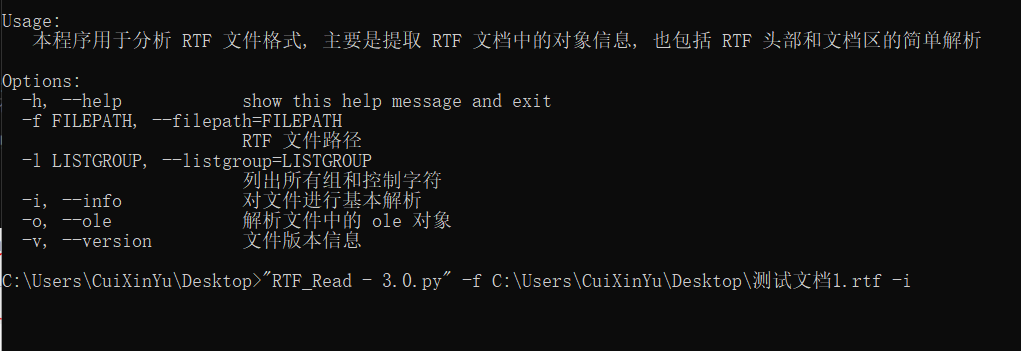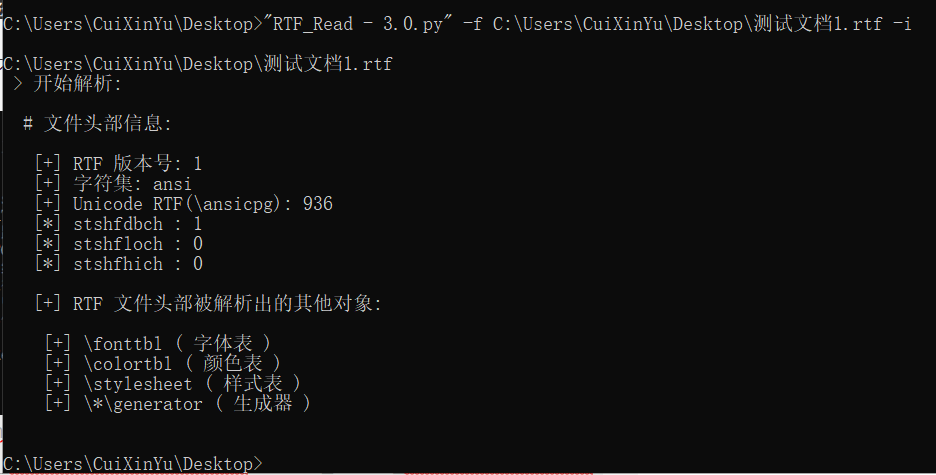c) 解析文件中的 ole 对象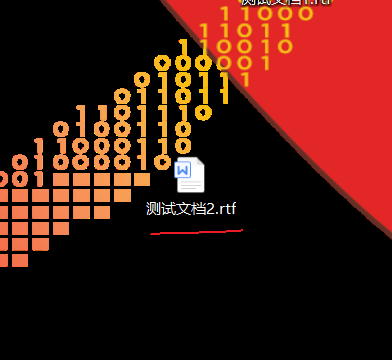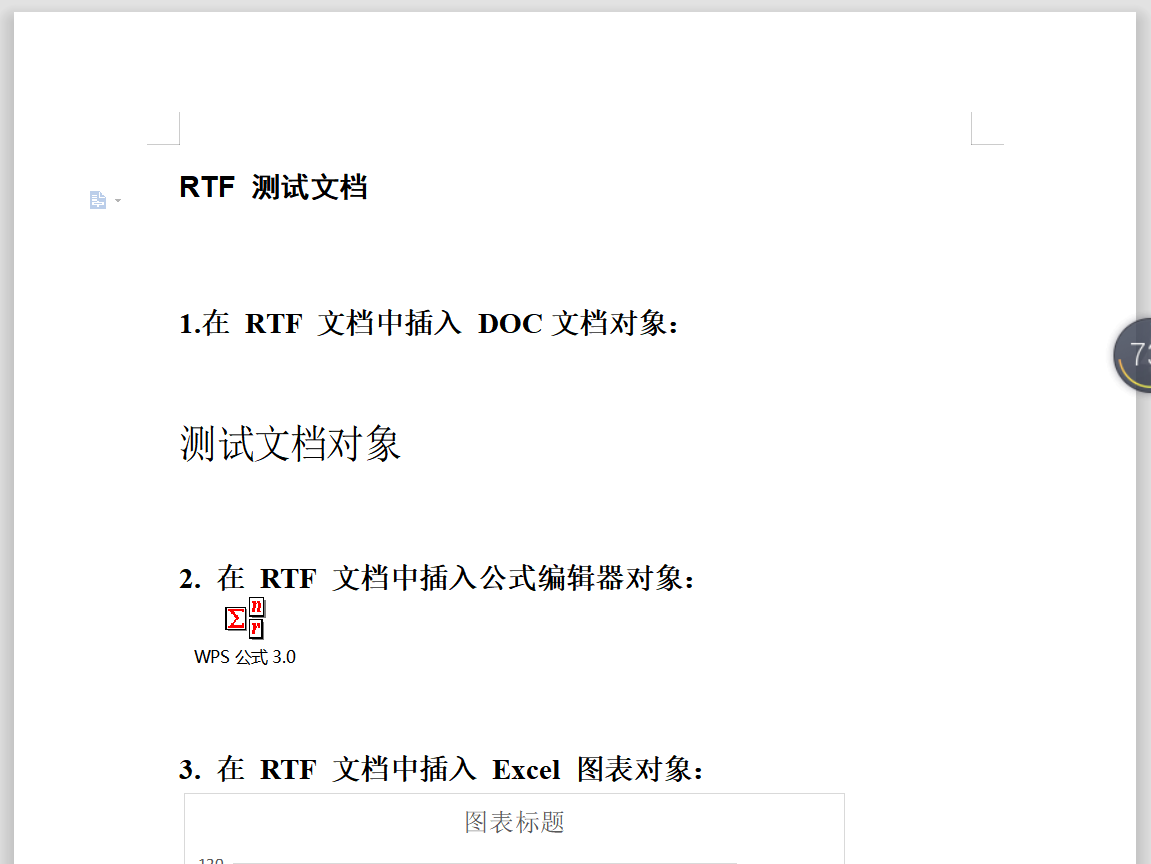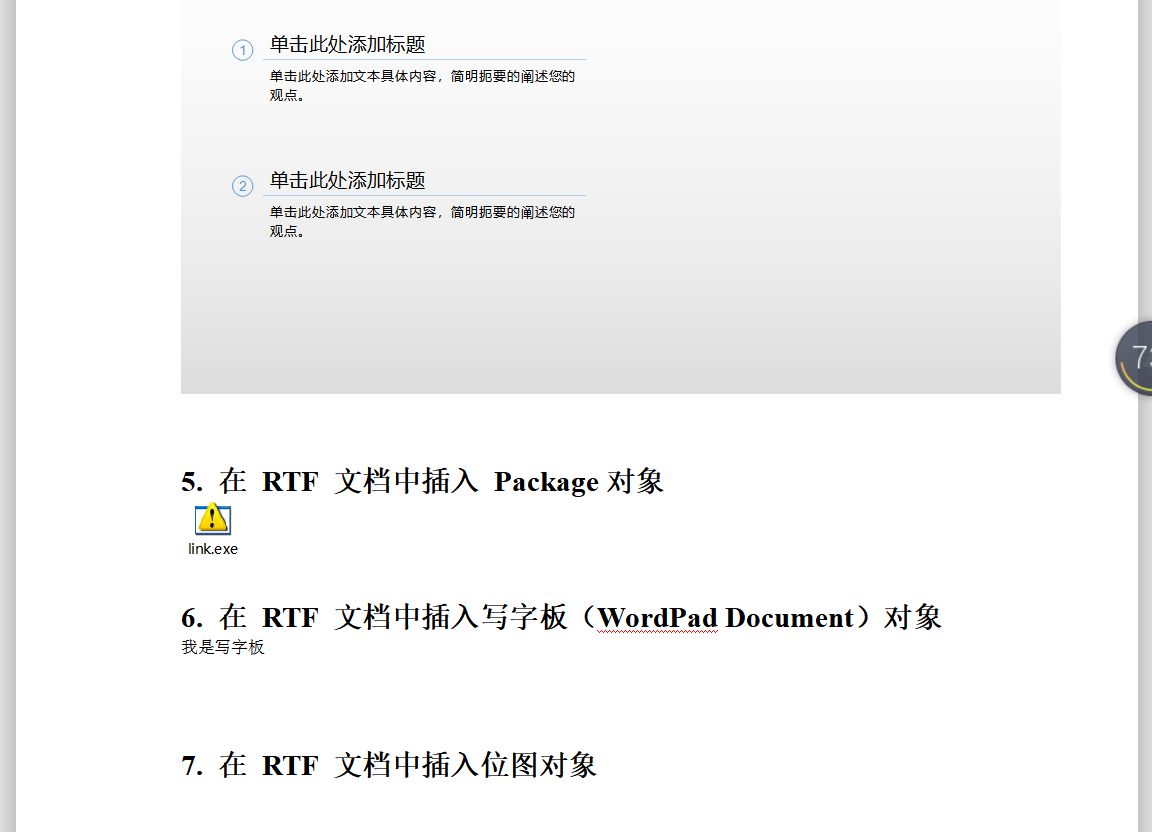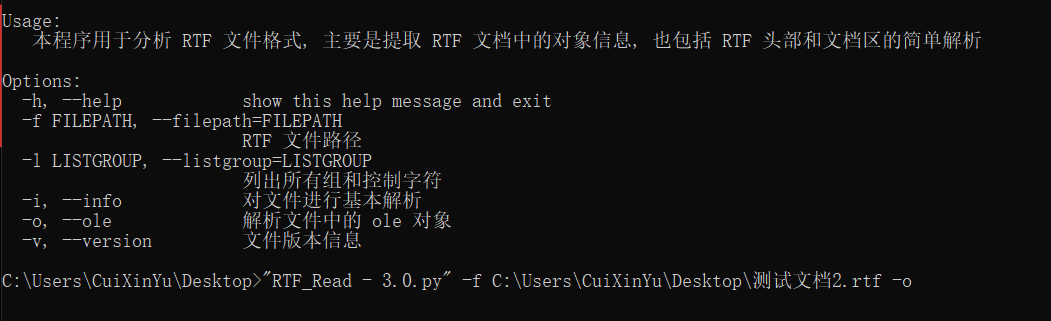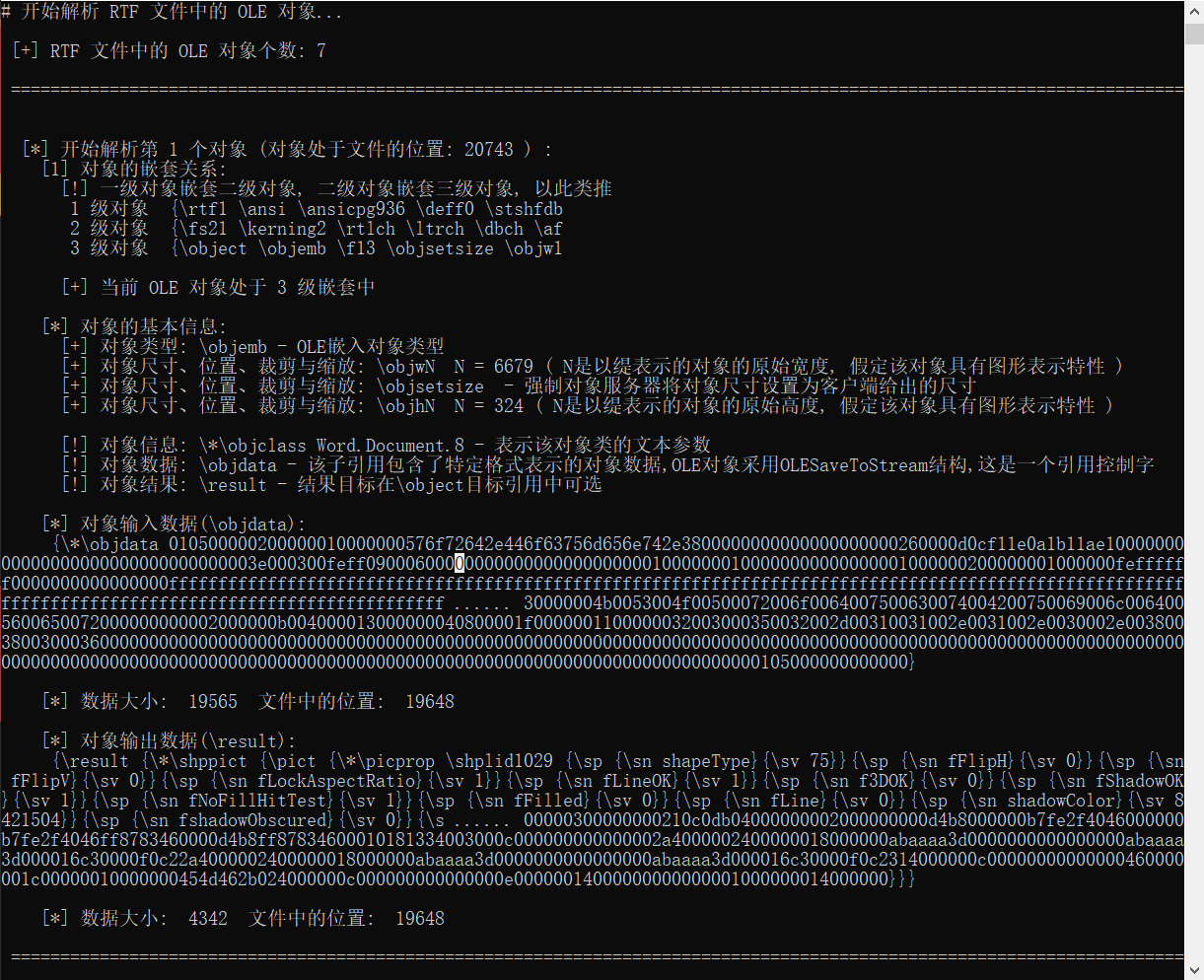– 第七个对象（最后一个）解析正常。